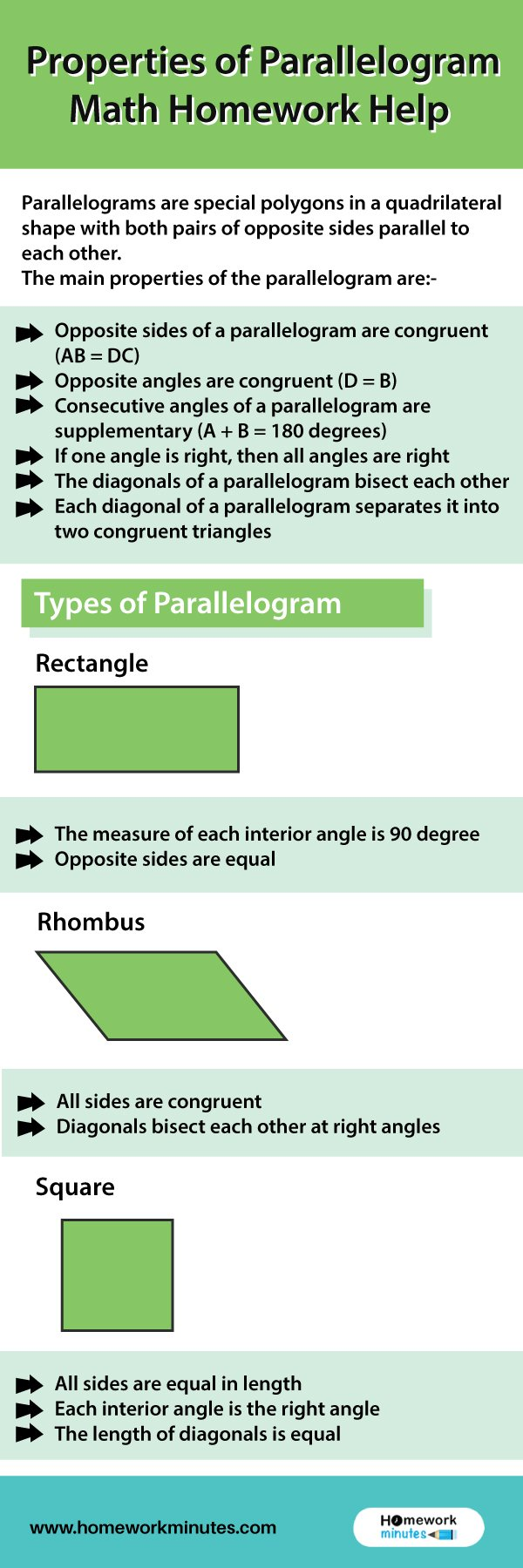# Properties of parallelogram | Math Homework HelpParallelograms are special polygons in a quadrilateral shape with both pairs of opposite sides parallel to each other. It is a 2D geometrical shape. A parallelogram is also a polygon having four sides with interior angles equal to each other. There are many 2D shapes and sizes present in geometry. For calculating the properties of a parallelogram, you need to find the base and height. In this guide, you’ll read the main properties of a parallelogram.

## Main Properties of Parallelogram

The main properties of the parallelogram are:-

• Opposite sides of a parallelogram are congruent (AB = DC)
• Opposite angles are congruent (D = B)
• Consecutive angles of a parallelogram are supplementary (A + B = 180 degrees)
• If one angle is right, then all angles are right
• The diagonals of a parallelogram bisect each other
• Each diagonal of a parallelogram separates it into two congruent triangles

For Example:-

ABCD is a parallelogram, where AB || CD and AD || BC.

Also, AB = CD and AD = BC

And, ∠A = ∠C & ∠B = ∠D

Also, ∠A & ∠D are supplementary angles because these interior angles lie on the same side of the transversal. Similarly, ∠B & ∠C are supplementary angles.

Therefore,

∠A + ∠D = 180

∠B + ∠C = 180

## Types of Parallelogram

There are mainly four types of Parallelograms as per distinct factors. Some of the key factors on which the types of parallelogram depends include angles, sides, etc.

For example:-

• In a parallelogram named ABCD

If AB = BC = CD= DA are equal, the type is known as a rhombus. Both rhombus and parallelogram have similar properties.

• The other two types of parallelograms are rectangular and square, respectively.

The other two special types of a parallelogram are:

To know about the main types in brief, here are these with basic properties.

### Rectangle

• A rectangle is a four-sided figure referred to as a quadrilateral
• The measure of each interior angle is a 90 degree
• Opposite sides are equal
• The sum of the interior angles of a rectangle is equal to 180
• The diagonals of a rectangle bisect each other

### Rhombus

• All sides are congruent
• Diagonals bisect each other at right angles
• The opposite sides of a rhombus are parallel
• The sum of two adjacent sides of a rhombus is 180
• There are no circumscribing or inscribing circles within or around a rhombus
• The opposite angles of a rhombus are equal

### Square

• All four interior angles of a square are equal to 90°
• All four sides of the square are congruent or equal to each other
• The opposite sides of the square are parallel to each other
• The diagonals of the square bisect each other at 90°
• The two diagonals of the square are equal to each other
• The square has four vertices and four sides
• The diagonal of the square divide it into two similar isosceles triangles
• The length of diagonals is greater than the sides of the square

If you need help with these typical mathematics topics, you can seek instant help from us. We are available 24*7 to provide you instant service. Chat with us now!

### Related Questions

Town B-Describe the Changes in Weather

Bullco Blends Silicon and Nitrogen To Produce Two Types of Fertilizers